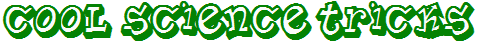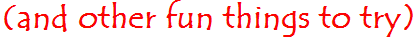Can you fold your tongue into a U shape? There is a certain gene which controls whether or not you can do this.Not everyone has that gene, so not everyone can do it!How to "Prove" that 2 Equals 1

 First let's refresh our memories on some basic math facts. Reminder #1: A number divided by itself equals one, as in these two examples.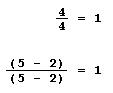Reminder #2: If we have an equation, we can multiply the same number (such as 2) on both sides of the equation.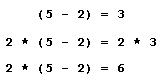Reminder #3: We can divide both sides of an equation by the same amount.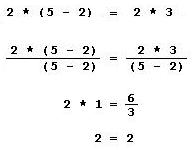Now let's use those basic math facts to "prove" that 2 = 1. Step A: If we have an equation, we can multiply the same number (such as 2) on both sides of the equation. See Reminder #2.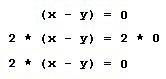Step B: Notice that the first equation and the third equation shown in Step A are both equal to the same value. Therefore, they are equal to each other.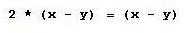Step C: We can divide both sides of an equation by the same amount. See Reminder #3. So apparently 2 equals 1!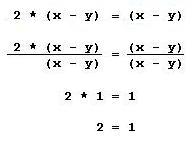Hanger Gong

 Hang a coat hanger onto a piece of string or yarn. Hold the ends of the string in your ears, and bend over so that the hanger isn't touching you. Carefully bang the hanger against various objects, or have someone tap the hanger with various objects. The sound waves travel up the string, and they sound like a loud gong in your ears!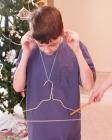Flag Illusion

 Stare at the white dot in the middle of the flag for 20 or 30 seconds. Then look at a white wall or ceiling. Where did the red, white, and blue come from?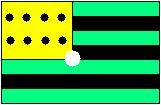Is it true, or is it a lie?

 If the sentence on the right is true, then it's a lie! If the sentence on the right is a lie, then it's true! Is it true, or is it a lie? "This sentence is a lie!"

"Cool Science Tricks"
URL: http://daveroot.neocities.org/sciencetricks/Page6.htm

Modification History
 01/18/2006: Added "Is it true, or is it a lie?" 01/04/2006: New page.

Dave Root